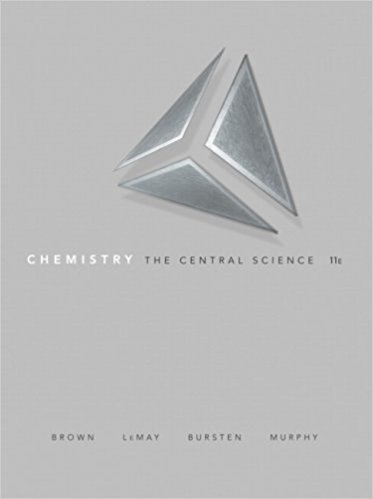×

×

Textbooks / Chemistry / Chemistry: The Central Science 11

# Chemistry: The Central Science 11th Edition - Solutions by Chapter## Full solutions for Chemistry: The Central Science | 11th Edition

ISBN: 9780136006176Chemistry: The Central Science | 11th Edition - Solutions by Chapter

Solutions by Chapter
4 5 0 418 Reviews
##### ISBN: 9780136006176

The full step-by-step solution to problem in Chemistry: The Central Science were answered by , our top Chemistry solution expert on 03/09/18, 07:03PM. Since problems from 25 chapters in Chemistry: The Central Science have been answered, more than 37468 students have viewed full step-by-step answer. Chemistry: The Central Science was written by and is associated to the ISBN: 9780136006176. This textbook survival guide was created for the textbook: Chemistry: The Central Science , edition: 11. This expansive textbook survival guide covers the following chapters: 25.

Key Chemistry Terms and definitions covered in this textbook

A reaction in which two atoms or groups of atoms react with a double bond, forming a compound with the two new groups bonded to the carbons of the original double bond.

• aldaric acid

A dicarboxylic acid that is produced when an aldose or ketose is treated with a strong oxidizing agent, such as HNO3.

• alkyl group

A substituent lacking p bonds and comprised of only carbon and hydrogen atoms.

• alkylation

A reaction that achieves the installation of an alkyl group. For example, an SN2 reaction in which an alkyl group is connected to an attacking nucleophile.

• bar

A unit of pressure equal to 105 Pa. (Section 10.2)

• bimolecular

For mechanisms, a step that involves two chemical entities.

• crossed Claisen condensation

A Claisen condensation reaction that occurs between different partners.

• Dalton’s law of partial pressures

A law stating that the total pressure of a mixture of gases is the sum of the pressures that each gas would exert if it were present alone. (Section 10.6)

• Dehydration

Elimination of water.

• heterogeneous catalyst

A catalyst that does not dissolve in the reaction medium.

• Hydroxyl group

An !OH group

• ion

Electrically charged atom or group of atoms (polyatomic ion); ions can be positively or negatively charged, depending on whether electrons are lost (positive) or gained (negative) by the atoms. (Section 2.7)

• molecular-orbital diagram

A diagram that shows the energies of molecular orbitals relative to the atomic orbitals from which they are derived; also called an energy-level diagram. (Section 9.7)

• oligomers

During the polymerization process, compounds constructed from just a few monomers.

• Polarimeter

An instrument for measuring the ability of a compound to rotate the plane of plane-polarized light.

• reaction quotient (Q)

The value that is obtained when concentrations of reactants and products are inserted into the equilibrium expression. If the concentrations are equilibrium concentrations, Q = K; otherwise, Q ? K. (Section 15.6)

• Resonance in NMR spectroscopy

The absorption of electromagnetic radiation by a precessing nucleus and the resulting “fl ip” of its nuclear spin from the lower energy state to the higher energy state.

• salt

An ionic compound formed by replacing one or more hydrogens of an acid by other cations. (Section 4.3)

• substrate

The starting alkyl halide in a substitution or elimination reaction.

• Vibrational infrared region

A common type of spin-spin coupling involving the H atoms on two C atoms that are bonded to each other.